# How to

17 Oct 201813 minutes to read

## Render Autocomplete from Code behind

Autocomplete can be rendered from the code behind by initializing the required properties in controller and passing those properties via ViewData or Model to the client side.

The following code illustrates the initialization of Autocomplete properties in the controller.

• C#
• ``````//Namespace to get the JavaScript (Autocomplete) component properties
using Syncfusion.JavaScript.Models;

namespace MvcApplication.Controllers
{
public class AutocompleteController : Controller
{
// GET: Autocomplete
public ActionResult AutocompleteFeatures()
{
//Initializing the Autocomplete model

AutocompleteProperties AutoObj = new AutocompleteProperties();

//Initializing the datasource and other properties

AutoObj.DataSource = "http://js.syncfusion.com/ejServices/wcf/NorthWind.svc/";
AutoObj.Query = "ej.Query().from('Suppliers').select('SupplierID', 'ContactName')";
AutoObj.AutocompleteFields.Text = "ContactName";
AutoObj.AutocompleteFields.Key = "SupplierID";
AutoObj.Width = "500px";
AutoObj.ShowPopupButton = true;

//Passing Autocomplete properties using the ViewData
ViewData["AutoModel"] = AutoObj;
return View();
}
}
}``````

Binding the Autocomplete properties passed via ViewData from the controller in the client side as below.

• CSHTML
• ``````@{
Html.EJ().Autocomplete("searchCustomer",(Syncfusion.JavaScript.Models.AutocompleteProperties)ViewData["AutoModel"]).Render();
}``````

## How to set autocomplete default value

You can set a value into autocomplete at initial rendering using `Value` property. It is used to select a single value from the autocomplete widget at initial rendering state.

Refer to the following code.

``@Html.EJ().Autocomplete("selectState").Datasource((IEnumerable<states>)ViewBag.datasource).AutocompleteFields(field => field.Key("index").Text("countryName")).Value("Arizona")``
``````public partial class AutocompleteController : Controller{
List<states> state = new List<states>();
public ActionResult AutocompleteFeatures()
{
state.Add(new states { index = "s1", countryName = "Alabama" });
state.Add(new states { index = "s3", countryName = "Arizona" });
ViewBag.datasource = state;
return View();
}
}
public class states
{
public string index { get; set; }
public string countryName { get; set; }
}``````

The following output is displayed as a result of the previous code example.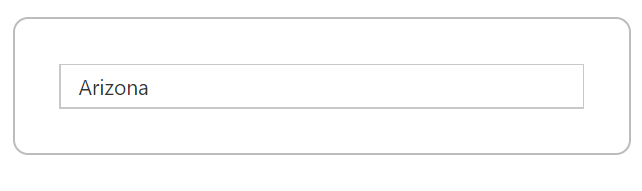If you set autocomplete `Value` property as a string that is present in the data source, the hidden input value holds the corresponding `Key` value. This is used for validation purpose in the autocomplete. For example, ‘Arizona’ text holds the key value of ‘s3’. The autocomplete default value ‘Arizona’ is present in the ‘countryName’ of data source and also this field is mapped for autocomplete `Text` property. So, hidden input value holds the ‘s3’ value.

The following screenshot illustrates the previous hidden input state of code.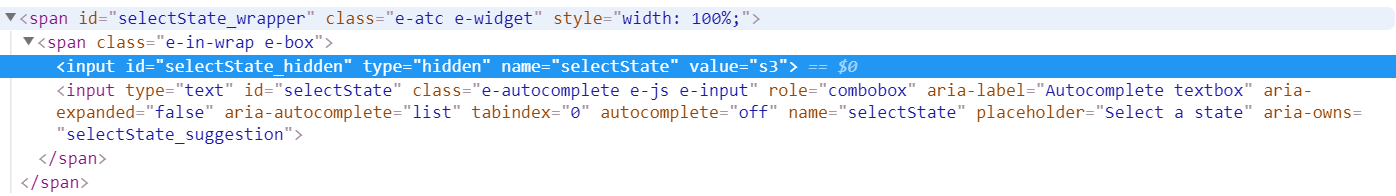If you set autocomplete `Value` property as a string that is not present in the data source, the hidden input value holds the autocomplete value. For example, if autocomplete default value is ‘New York’ and not present in the ‘countryName’ of data source, the hidden input value holds the ‘New York’string.

Refer to the following code sample.

• RAZOR
• ``@Html.EJ().Autocomplete("selectState").Datasource((IEnumerable<states>)ViewBag.datasource).AutocompleteFields(field => field.Key("index").Text("countryName")).Value("New York")``

The following screenshot illustrates the previous hidden input state of code.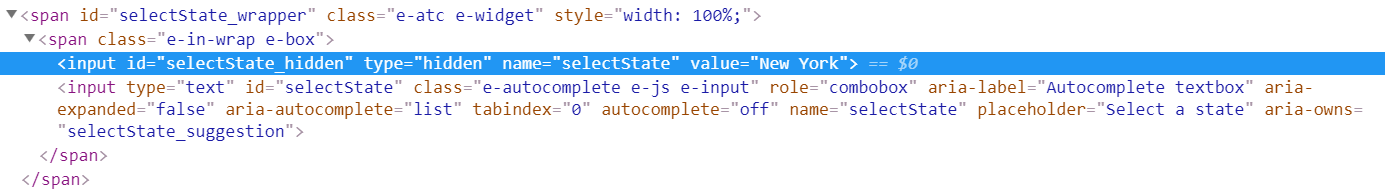### Remote data

The remote data also allows you to set the default value into autocomplete using `Value` property.

Refer to the following code sample.

``@Html.EJ().Autocomplete("selectCar").Datasource(dataSource => dataSource.URL(Url.Action("GetOutletsForAutocomplete", "Autocomplete")).Adaptor(AdaptorType.UrlAdaptor)).AutocompleteFields(field => field.Text("Name").Key("Id")).Value("Two")``
``````public ActionResult GetOutletsForAutocomplete(DataManager dm)
{
var models = GetTestData();

IEnumerable Data = GetTestData();
Syncfusion.JavaScript.DataSources.DataOperations operation = new Syncfusion.JavaScript.DataSources.DataOperations();

var data = operation.Execute(models, dm);
if (dm.Where != null && dm.Where.Count > 0) //Filtering
{
data = operation.PerformWhereFilter(models, dm.Where, dm.Where.Operator);
}
return Json(data, JsonRequestBehavior.AllowGet);
}

public class AutocompleteModel
{
[Display(Name = "Id")]
public string Id { get; set; }
[Display(Name = "Name")]
public string Name { get; set; }
}

private List<AutocompleteModel> GetTestData()
{
var list = new List<AutocompleteModel>();
list.Add(new AutocompleteModel(){ Id = "1", Name = "One"});
list.Add(new AutocompleteModel(){ Id = "2", Name = "Two"});
list.Add(new AutocompleteModel(){ Id = "3", Name = "Three"});
return list;
}``````

The autocomplete `Value` property triggers a server-side post when using remote data in the autocomplete. The server side data manger holds `where` query which contains field `name` as autocomplete `Text` property.

Find the following screenshot for the data manager where query.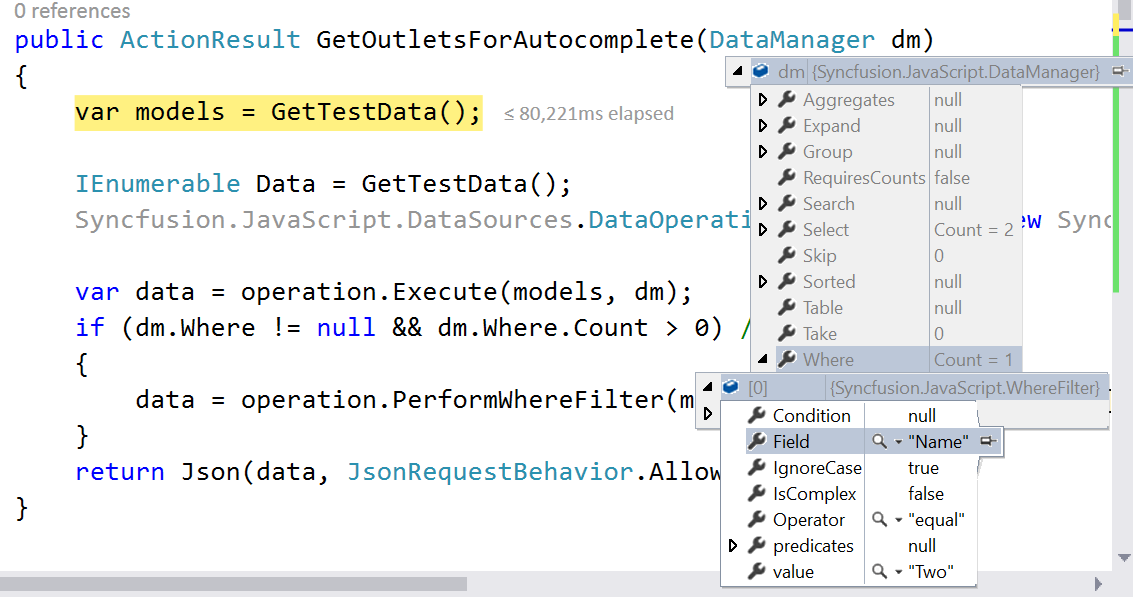Find the following output for the previous code.## How to show text on autocomplete using key value

You can set the value of autocomplete text box based on a given key value. The `SelectValueByKey` property is used to select autocomplete value based on the specified key value.

Refer to the following code sample.

``@Html.EJ().Autocomplete("selectState").Datasource((IEnumerable<states>)ViewBag.datasource).AutocompleteFields(field => field.Key("index").Text("countryName")).SelectValueByKey("s2")``
``````public partial class AutocompleteController : Controller{
List<states> state = new List<states>();
public ActionResult AutocompleteFeatures()
{
state.Add(new states { index = "s1", countryName = "Alabama" });
state.Add(new states { index = "s3", countryName = "Arizona" });
ViewBag.datasource = state;
return View();
}
}
public class states
{
public string index { get; set; }
public string countryName { get; set; }
}``````

For example, If `SelectValueByKey` property specified the value as ‘s2’, the corresponding `Text` value `Alaska` is shown in the autocomplete text.

The following output is displayed as a result of the previous code example.If you are specifying the `SelectValueByKey` property into autocomplete control, the hidden input value holds a specified key value.

Refer to the following screenshot.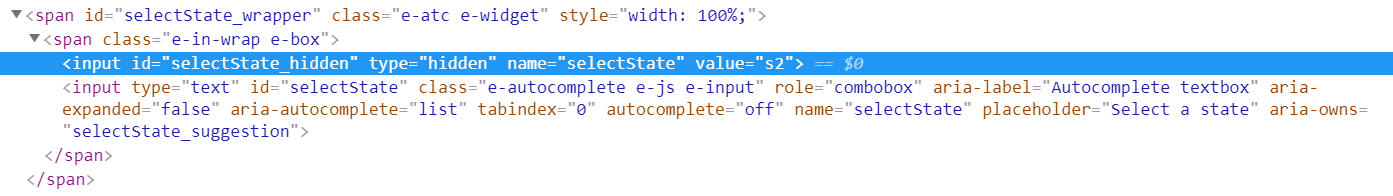### Remote data

The remote data also allows you to set the default value into autocomplete based on a given key value using the `SelectValueByKey` property.

Refer to the following code snippet.

``@Html.EJ().Autocomplete("selectCar").Datasource(dataSource => dataSource.URL(Url.Action("GetOutletsForAutocomplete", "Autocomplete")).Adaptor(AdaptorType.UrlAdaptor)).AutocompleteFields(field => field.Text("Name").Key("Id")).SelectValueByKey("2")``
``````public ActionResult GetOutletsForAutocomplete(DataManager dm)
{
var models = GetTestData();

IEnumerable Data = GetTestData();
Syncfusion.JavaScript.DataSources.DataOperations operation = new Syncfusion.JavaScript.DataSources.DataOperations();

var data = operation.Execute(models, dm);
if (dm.Where != null && dm.Where.Count > 0) //Filtering
{
data = operation.PerformWhereFilter(models, dm.Where, dm.Where.Operator);
}
return Json(data, JsonRequestBehavior.AllowGet);
}

public class AutocompleteModel
{
[Display(Name = "Id")]
public string Id { get; set; }
[Display(Name = "Name")]
public string Name { get; set; }
}

private List<AutocompleteModel> GetTestData()
{
var list = new List<AutocompleteModel>();
list.Add(new AutocompleteModel(){ Id = "1", Name = "One"});
list.Add(new AutocompleteModel(){ Id = "2", Name = "Two"});
list.Add(new AutocompleteModel(){ Id = "3", Name = "Three"});
return list;
}``````

The autocomplete `SelectValueByKey` property triggers a server-side post when using remote data on autocomplete. The server side data manger holds `where` query which contains field `name` as autocomplete `Key` property.

Find the following screenshot for the data manager where query.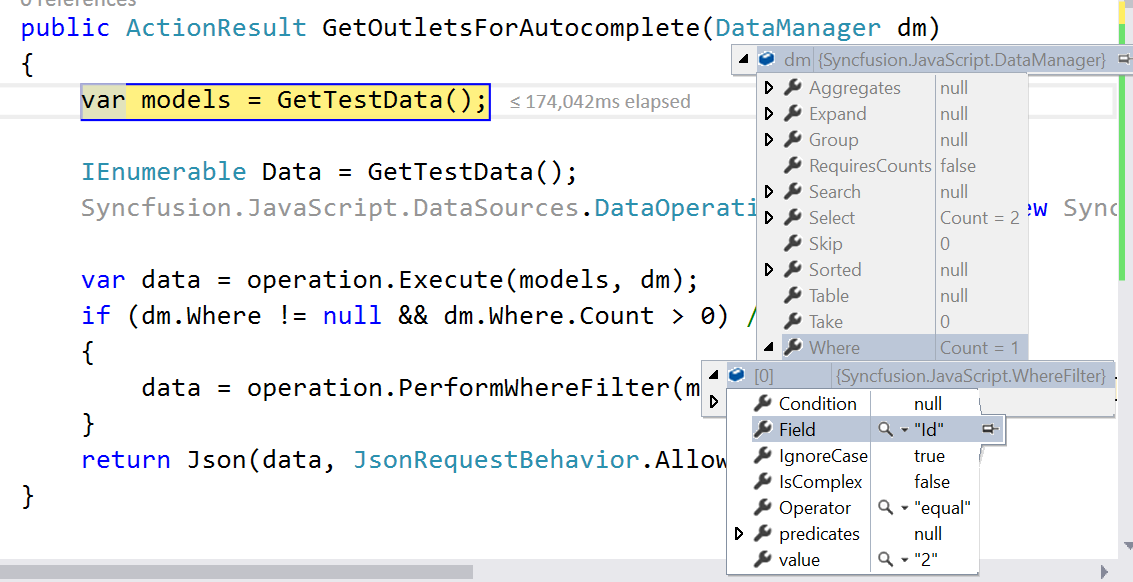Find the output for the previously given code as follows.